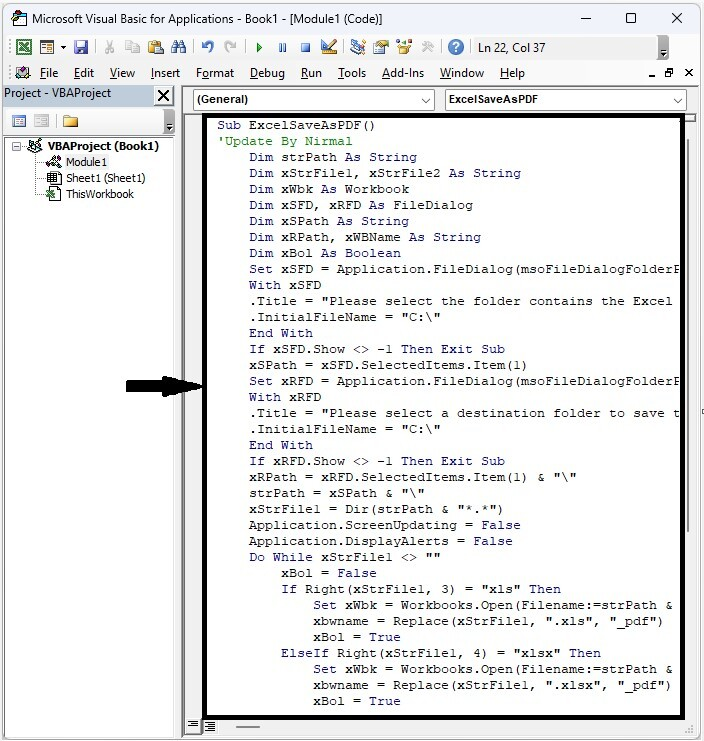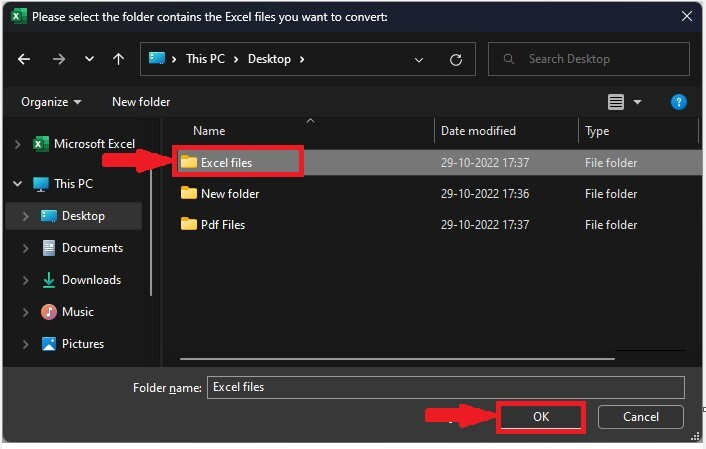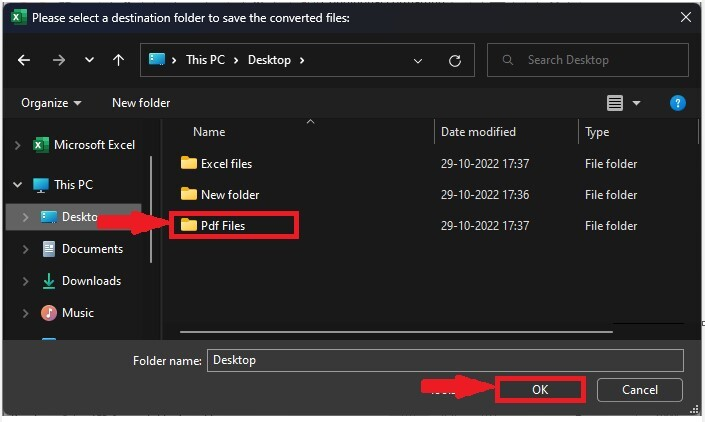# How to Convert Multiple Workbooks or Worksheets to PDF Files at Once in Excel?

Sometimes, while working in Excel, you have needed to convert an Excel workbook to PDF. It can be a time-consuming process if you try to do this manually. We can complete the task using the VBA application as it can’t be completed directly in excel. Read this article to learn how you can convert multiple workbooks or worksheets to PDF files at once in Excel. Let us see the procedure in a briefer way.

## Converting Multiple Workbooks to PDF Files at Once in Excel

Here we will first create a VBA module, then run it to select folders that contain workbooks and PDFs, then click OK to complete the task. Let us see a simple process to know how we can convert multiple workbooks to PDF files once they are in Excel.

### Step 1

Let us consider a new Excel sheet, then right-click on the sheet name and select view code to open the vba application, then click on inset and select module.

Right click > View code > Inset > Module

Then, as shown in the image below, type the following program code into the text box.

### Program 1

Sub ExcelSaveAsPDF()
'Update By Nirmal
Dim strPath As String
Dim xStrFile1, xStrFile2 As String
Dim xWbk As Workbook
Dim xSFD, xRFD As FileDialog
Dim xSPath As String
Dim xRPath, xWBName As String
Dim xBol As Boolean
Set xSFD = Application.FileDialog(msoFileDialogFolderPicker)
With xSFD
.Title = "Please select the folder contains the Excel files you want to convert:"
.InitialFileName = "C:"
End With
If xSFD.Show <> -1 Then Exit Sub
xSPath = xSFD.SelectedItems.Item(1)
Set xRFD = Application.FileDialog(msoFileDialogFolderPicker)
With xRFD
.Title = "Please select a destination folder to save the converted files:"
.InitialFileName = "C:"
End With
If xRFD.Show <> -1 Then Exit Sub
xRPath = xRFD.SelectedItems.Item(1) & ""
strPath = xSPath & ""
xStrFile1 = Dir(strPath & "*.*")
Application.ScreenUpdating = False
Do While xStrFile1 <> ""
xBol = False
If Right(xStrFile1, 3) = "xls" Then
Set xWbk = Workbooks.Open(Filename:=strPath & xStrFile1)
xbwname = Replace(xStrFile1, ".xls", "_pdf")
xBol = True
ElseIf Right(xStrFile1, 4) = "xlsx" Then
Set xWbk = Workbooks.Open(Filename:=strPath & xStrFile1)
xbwname = Replace(xStrFile1, ".xlsx", "_pdf")
xBol = True
ElseIf Right(xStrFile1, 4) = "xlsm" Then
Set xWbk = Workbooks.Open(Filename:=strPath & xStrFile1)
xbwname = Replace(xStrFile1, ".xlsm", "_pdf")
xBol = True
End If
If xBol Then
xWbk.ExportAsFixedFormat Type:=xlTypePDF, Filename:=xRPath & xbwname & ".pdf"
xWbk.Close SaveChanges:=False
End If
xStrFile1 = Dir
Loop
Application.ScreenUpdating = True
End Sub### Step 2

Then save the sheet as a macro-enabled workbook, select the folder where the excel files are present, and click OK.### Step 3

Now select the folder where you want to store your PDF files and click OK to complete our process.This is how we can convert multiple workbooks to PDF files at once in Excel.

If we need to convert multiple worksheets from a single workbook, we use Program 2 after opening the workbook.

### Program 2

Sub SplitEachWorksheet()
'Update by Nirmal
Dim xSPath As String
Dim xSFD As FileDialog
Dim xWSs As Sheets
Dim xWb As Workbook
Dim xWbs As Workbooks
Dim xNWb As Workbook
Dim xInt, xI As Integer
Set xSFD = Application.FileDialog(msoFileDialogFolderPicker)
With xSFD
.title = "Please select a folder to save the converted files:"
.InitialFileName = "C:"
End With
If xSFD.Show <> -1 Then Exit Sub
xSPath = xSFD.SelectedItems.Item(1)
Application.ScreenUpdating = False
Set xWb = Application.ActiveWorkbook
Set xWbs = Application.Workbooks
Set xWSs = xWb.Sheets
xInt = xWSs.Count
For xI = 1 To xInt
On Error GoTo EBreak
Set xWs = xWSs.Item(xI)
If xWs.Visible Then
xWSs(xWs.Name).Copy
Application.ActiveSheet.ExportAsFixedFormat Type:=xlTypePDF, Filename:=xSPath & "" & xWs.Name & ".pdf"
Application.ActiveWorkbook.Close False
End If
EBreak:
Next
xWb.Activate